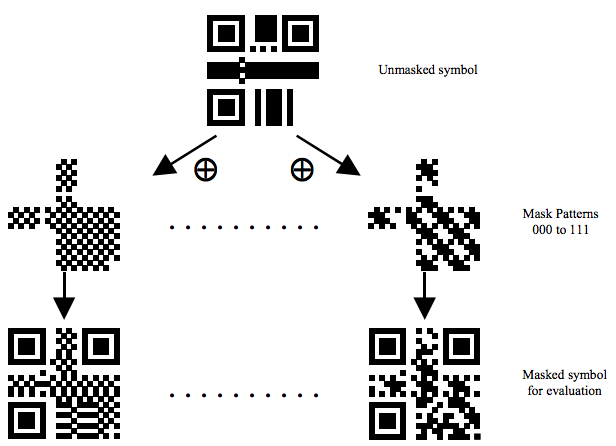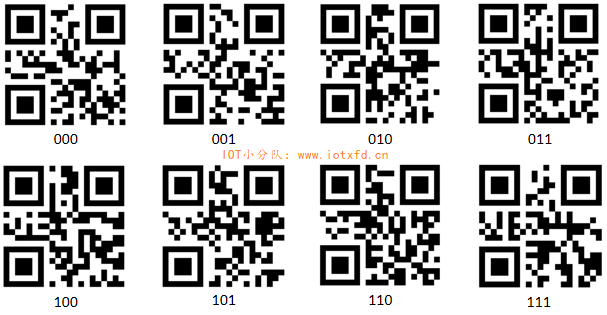Categories

# Detailed QR code (lower)

Fast response code (lower)

Book connected to the back, to continue in the second half.

Error correction code

QR code series generated using error correction algorithm error correction code word, is added after the data code word sequence so that symbols can be recovered in the event of damage. This is why the two-dimensional code even if they are defective can be swept out. Without any defects, we should also create incomplete sweep it out, I believe we have seen a lot of the middle belt icons of two-dimensional bar code.

Error correction code word can correct both types of errors, NoRead error (error code word location is known) and alternate error (error code word location is unknown). NoRead a mistake is not a scan or not to decode the symbol characters, a symbol character substitution error is wrong coding. When a defective module becomes so dark light module, the module is dark or light module, the symbol characters are erroneously decoded to a different code words, resulting in substitution errors, errors such data requires alternative two error correction code words to correct.

Error correction level

There are four levels of error correction, the error correction capacity corresponding to four kinds shown in the following table.

Error correction level

Error correction capacity,% (approximate)

L M Q H
7 15 25 30

Users should determine the appropriate level of error correction to meet the application requirements. Capacity detection and correction from L to H to provide four different levels are gradually increased at the cost of a given size representing the data symbol length gradually increases. For example, a version of the 20-Q symbol data can contain codewords 485, if error correction can accept a lower level, the same data may also be version 15-L symbolic representation (accurate data capacity of 523 yards word).

Select the error correction level associated with the following factors:

The quality level is expected to sign: the sign is expected to lower the quality grade, the higher the error correction level should be applied.

The importance of the first reading rate.

After scanning misreading failed again scanning opportunities.

Space limits the use of printed symbols higher error correction level.

[L] level error correction symbol has a suitable quality and / or to the symbol indicates that the required data is given the smallest possible case. [M] level is considered the “standard” level, it has a smaller size and higher reliability. [Q] having a grade level “high reliability”, applicable to some important difference symbol printing quality or the case, [H] level to provide the highest reliability can be achieved.

Error correction code word generated

Error correction using QR codes of Reed-Solomon codes, Reed-Solomon code related, you can refer to this article: http: //article.iotxfd.cn/RFID/Reed%20Solomon%20Codes. Here I can only outline the calculation process.

Error correction code word generating polynomial

Error correction code word error correction code is the remainder polynomial obtained in addition to data codeword. Error correction code polynomial we can look-up table come, first check the following table 3: QR code symbol versions of error correction features. Here I list only a small part of the complete data please see table GB / T 18284-2000 Table 9.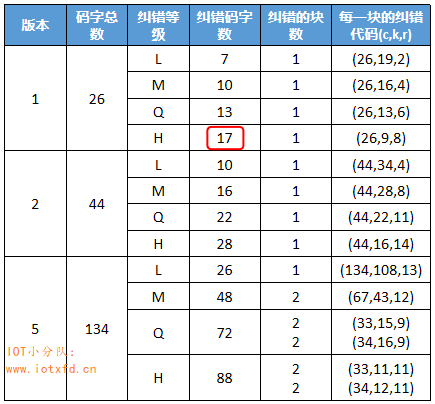Wherein (c, k, r): c = the total number of codewords; k = data code words; r = the error correction capacity.

Before  Continued Example 1 determined using version 1-H, look-up table to obtain an error correction code words: 17 (Table red frame portion). Total number of code word 26 indicates the total data amount QR code versions can be accommodated, wherein the data representing the codeword 9, 17 error correction code word account. Then according to the error correction code words 17 to find a polynomial. Can be a generator polynomial error correction code word table in Appendix A of GB / T 18284-2000 find, using the generator polynomial tool may create it, are listed in Table 4 only a small portion: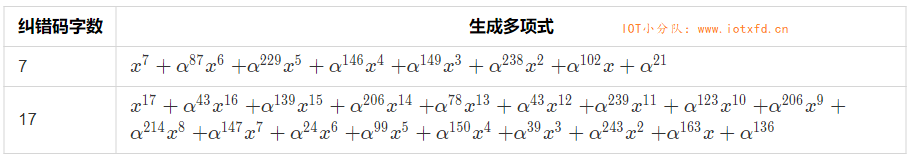Reed-Solomon code in C #

You might ask, previously generated error correction code word how with this polynomial except ah? Direct addition is certainly not, first of all to be found in polynomial converted to the corresponding set of numbers. Found in the table 17 corresponding to the generating polynomial can be converted to: [1, 119, 66, 83, 120, 119, 22, 197, 83, 249, 41, 143, 134, 85, 53, 125, 99, 79 ]. This is obtained by dividing the remainder set of digital data code word is our word of the error correction code. Of course, this process is using the program to complete. Reed-Solomon coding This article describes in detail how to use Python to achieve this function. I will need to use the code translated into C #:

``````using System;

namespace QRHelper
{
class ECC
{
const int PRIM = 0x11d;

private static byte[] gfExp = new byte; //逆对数(指数)表
private static byte[] gfLog = new byte; //对数表

static ECC()
{
byte x = 1;
for (int i = 0; i <= 255; i++)
{
gfExp[i] = x;
gfLog[x] = (byte)i;
x = Gf_MultNoLUT(x, 2);
}

for (int i = 255; i < 512; i++)
{
gfExp[i] = gfExp[i - 255];
}
}

//伽罗华域乘法
private static byte Gf_MultNoLUT(int x, int y)
{
int r = 0;
while (y != 0)
{
if ((y & 1) != 0)
{
r ^= x;
}
y >>= 1;
x <<= 1;
if ((x & 256) != 0)
{
x ^= PRIM;
}
}
return (byte)r;
}

//伽罗华域乘法
private static byte GfMul(byte x, byte y)
{
if (x == 0 || y == 0)
{
return 0;
}
return gfExp[gfLog[x] + gfLog[y]];
}

//伽罗华域幂
private static byte GfPow(byte x, int power)
{
return gfExp[(gfLog[x] * power) % 255];
}

//多项式 乘法
private static byte[] GfPolyMul(byte[] p, byte[] q)
{
byte[] r = new byte[p.Length + q.Length - 1];
for (int j = 0; j < q.Length; j++)
{
for (int i = 0; i < p.Length; i++)
{
r[i + j] ^= GfMul(p[i], q[j]);
}
}
return r;
}

///
/// 获取纠错码字的生成多项式
///
/// 纠错码字数
/// 由一组数字表示的生成多项式
public static byte[] RsGeneratorPoly(int nsym)
{
byte[] g = { 1 };
for (int i = 0; i < nsym; i++)
{
g = GfPolyMul(g, new byte[] { 1, GfPow(2, i) });
}
return g;
}

///
/// 生成纠错码，并添加在数据码字之后
///
/// 数据码字
/// 纠错码字数
/// 数据码字+纠错码字
public static byte[] RsEncodeMsg(byte[] msgIn, int nsym)
{
if (msgIn.Length + nsym > 255)
{
throw new ArgumentException("数组长度超过 255！");
}
//byte[] gen = generators[(byte)nsym];
byte[] gen = RsGeneratorPoly(nsym);
byte[] msgOut = new byte[msgIn.Length + gen.Length - 1];
Array.Copy(msgIn, 0, msgOut, 0, msgIn.Length);

for (int i = 0; i < msgIn.Length; i++)
{
byte coef = msgOut[i];
if (coef != 0)
{
for (int j = 1; j < gen.Length; j++)
{
msgOut[i + j] ^= GfMul(gen[j], coef);
}
}
}
Array.Copy(msgIn, 0, msgOut, 0, msgIn.Length);

return msgOut;
}
}
}``````

The amount of code is quite small ah! According to surf the Internet without algorithm package. In the actual development, if you need to draw a large number of QR codes, complete all 31 generating polynomial can be converted results stored in the collection, use direct query can be derived, which can greatly speed up the production rate. RsGeneratorPoly above code () method for generating polynomial, it will produce a large temporary array. With code, we can continue our previous example of.

[Example 2] 1 Continued: generating a complete code word

00010000,00100000,00001100,01010110,01100001,10000000,11101100,00010001,11101100

Hexadecimal representation as: 0x10, 0x20, 0x0C, 0x56, 0x61, 0x80, 0xEC, 0x11, 0xEC

Then use the following code to generate a complete codeword:

``````byte[] msgin = { 0x10, 0x20, 0x0C, 0x56, 0x61, 0x80, 0xEC, 0x11, 0xEC };
byte[] msg = ECC.RsEncodeMsg(msgin, 17);``````

Get results: 0x10 0x20 0x0C 0x56 0x61 0x80 0xEC 0x11 0xEC 0x0E 0x9D 0x02 0xC8 0xC2 0x94 0xF3 0xA7 0xAD 0x8D 0xE2 0x0A 0xF4 0xA5 0x2B 0xAC 0xDF

And that we have to fill in all the QR code pattern 26 yards a word. Data for the first nine code words, after 17 error correction code word, the program has helped us to automatically connect better.

The final code sequence construction information

In the embodiment, the number of blocks of only one correction, simply connecting the data code word error correction code word are connected to form a data to. In the majority version, there are a plurality of error correction code block number. The following explains a plurality of error correction code blocks off configuration.

In Example 5-H version, the version 3 of the look-up table portion 5, as follows:Version 5-H codeword is divided into 4, wherein the total number of word two yards 33, 11 comprises a data code word and error correction code word 22; the other two yards total number of word 34, data 12 including 22 code word and error correction code word. The first 11 codewords of data are removed first data codeword, calculation error correction code word 22, are connected to form a data block; then take 11 code words from the data generation block 2 codeword; continues to fetch data from the code word 12 codeword data generation block 3; the last 12 codewords of data are taken out and the data generation block 4.

Each block in the table of characters arranged, each row of the table corresponds a codeword data block (denoted as Dn) and error correction code word (expressed as En) of the corresponding block;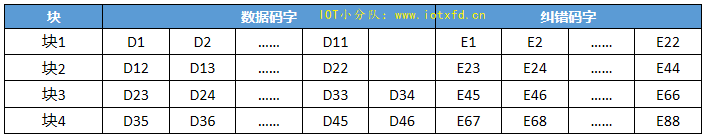The final version of the code sequence of symbols is 5-H:
D1, D12, D23, D35, D2, D13, D24, D36, ... D11, D22, D33, D45, D34, D46, E1, E23, E45, E67, E2, E24, E46, E68, ... E22, E44, E66, E88. In some versions, 3, 4 or 7 required in order to fill the remaining bit number of the coding region of the module, this time to be behind the last code word plus the remaining bits (0).

Format Information

Format used to store information error correction level and mask information, a data 15, the error correction by the indicator 2 + 3 + reference mask pattern 10 composed of an error correction code.

Calculated format information

First error correction indicator represented by 2 bits, each corresponding to the digital error correction level in Table 5 below.

Error correction level

Binary indicator

L M Q H
01 00 11 10

Reference mask pattern using three bits, represented by a number from 0 to 7, which can be converted to 3-bit binary mask later in the article, you need to know now occupy three bits on the line.

The error correction indicator 2 + 3 a mask pattern reference, to give 5-bit data codes, using BCH (15,5) encoding the calculated error correction code.

BCH code

BCH code and Reed-Solomon codes similar to Reed-Solomon encoding can refer to this article. Reed-Solomon code error correction code is derived using a polynomial division sequence, the BCH code is much simpler, it is an error correction code derived bitwise operation. BCH (15,5) BCH code represents a total length of 15 bits, wherein data symbols is 5 bits, the error correction code 10. Reed-Solomon code with a generator polynomial, BCH code is used to generate code: 10100110111. Using the data divided by the code generated code, the resulting remainder is the error correction code. Since the calculation of the BCH code is very simple, the following calculation process presentation data code of 00101.

The left data symbols 10, round 15, to give binary numbers: 001010000000000

The above number is divided 10100110111 (0x537), the use of long division, as shown below: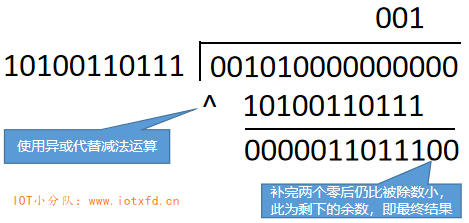The remainder is the above figure 10 takes the error correction code: 0011011100

The 5-bit data code 00101 is connected to an error correction code, to obtain a final format information code: 001010011011100 (0x14DC)

Program implementation is very simple to use, add the following code ECC class:

`````` //生成 BCH 码
private static int CheckFormat(int fmt)
{
int g = 0x537;
for (int i = 4; i >= 0; i--)
{
if ((fmt & (1 << (i + 10))) != 0)
{
fmt ^= g << i;
}
}
return fmt;
}

///
/// 生成 BCH(15,5) 纠错码，并返回完整格式信息码
///
/// 数据码
/// 返回完整格式信息码
public static int BCH_15_To_5_Encode(int data)
{
data <<= 10;
return data ^ CheckFormat(data);
}``````

With the following code format of the complete information code:

``int code = ECC.BCH_15_To_5_Encode(5);``

Results: 5340 (0x14DC)

To ensure that the reference error correction level and mask pattern (described later) together result not all 0, the format information 15 and the mask pattern 101010000010010 (0x5412) need to XOR.

Draw format information

QR codes have a special format information rendering region, as shown below: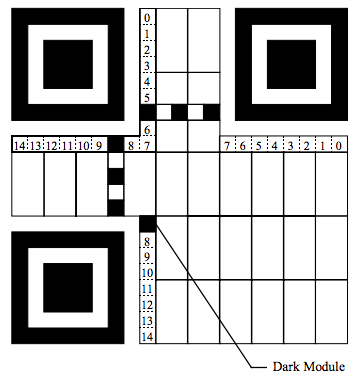Since correct decoding format of the information, it will be drawn twice to translate the entire essential symbol in the QR code to provide redundancy. Module lowest bit numbering format information is 0, the maximum number is 14 bits. To avoid confusion, the following table designates the respective bit number of the mask pattern generates format information and the results after both before performing the XOR operation.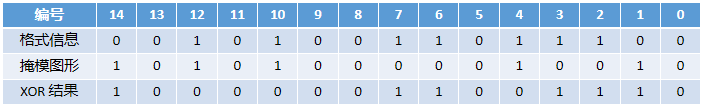Upper left corner of the drawing area number between 8,9 and dark modules 5, 6 are positioned between the graphics used, it is not used to draw the format information. Dark Module on the lower left corner number 8 is always a dark module is not used to store any information.

Next we will draw the XOR result of the format information region of the QR code, as shown below: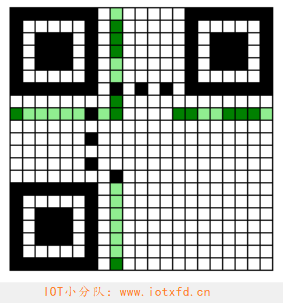The green area format information area, wherein the light module represents a pale green, dark green dark block represents.

Example 3  Continued: generating format information

Then I continued to [Example 1], add formatting information:

[Example 1] Before we chose the error correction level is H, look-up table 5, to give numbers: 10

Suppose we choose the reference mask pattern 011, the final data code: 10011

Using the previous procedure to generate full format information code 10011: 100110111000010 (0x4DC2)

1. 将生成的格式信息码与 101010000010010（0x5412）进行异或运算，结果为：
001100111010000（0x19D0）
2. The results are plotted in the format information area, the final results are shown below:Version Information

Version information for the version number stored QR code. Wherein the 6-bit data bits, 12 through BCH (18,6) encoding the calculated error correction bits. Only version symbol 7 to 40 contains the version information. Version 0 to 6 do not need to draw the version number.

Computing version information

Calculating format information and version information are similar, is the use of long division. Only this time using the generated code is: 1111100100101 (0x1F25). The following is a BCH (18,6) C # code:

``````public static int BCH_18_6_Encode(int data)
{
int g = 0x1F25;
int fmt = data << 12;
for (int i = 5; i >= 0; i--)
{
if ((fmt & (1 << (i + 12))) != 0)
{
fmt ^= g << i;
}
}
return (data << 12) ^ fmt;
}``````

Example 7 below to the version number, the version information code is calculated:

7 version number is converted to 6-bit binary data code: 000111

The above data symbols left 12, round 18: 000111000000000000

The above number is divided by the generated code 1111100100101 (0x1F25), to give the remainder: 110010010100

The data symbols are connected to the remainder obtained to give the final version information code: 000111110010010100

And different information formats, eliminating the need for a separate masking operation after code generation version information.

Draw version information

Since correct decoding version information is the key to the symbols correctly decoded, so the version information appears twice in the symbol to provide redundancy. A first storage position above the positioning pattern, a module 6 rows × 3 columns, whose right-close position detection pattern of the upper right corner of the separator; the second storage position in the left side of the finder pattern, which is close to the lower position of the lower left corner blue pattern detecting portion shown delimiters, the following figure: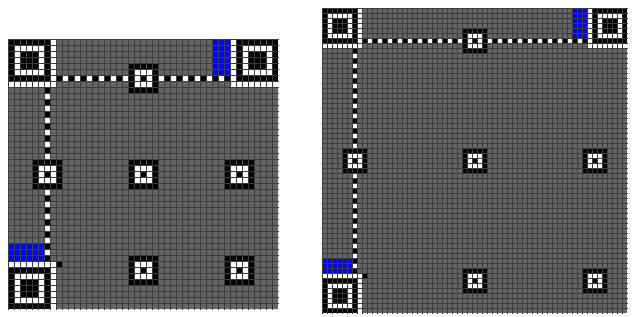Module lowest bit numbering format information is 0, the maximum number is 17 bits. Next we calculated the previous version information code to version 7 of the drawing area information QR code. Results as shown below, the red part of the version information, wherein the representative dark magenta module, the representative light pink module. :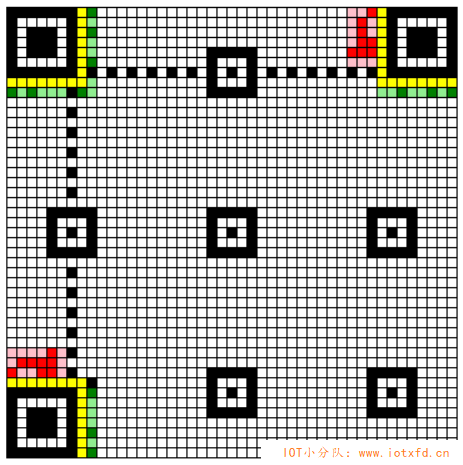So far, all the features of the graphics format graphics have been drawn and finished, and all have been shown in this picture above. Next, the data can finally start drawing a code word.

The arrangement of symbol characters

Coding region QR code symbol, the symbol characters in two modules wide columns arranged from the lower right corner of the symbol, and from right to left, and are alternately arranged from the top downwards or from the bottom upward. GB / T 18284-2000 with a long length coding to explain the layout rules, is actually very simple, is to be classified as units of two or arranged up and down, snake-like walk in the column, obstacles encountered skipped. For the convenience of everyone to learn, I joined the line drawing functions take place in the "QR Assistant Program", below is the route to walk version 1 and version 7: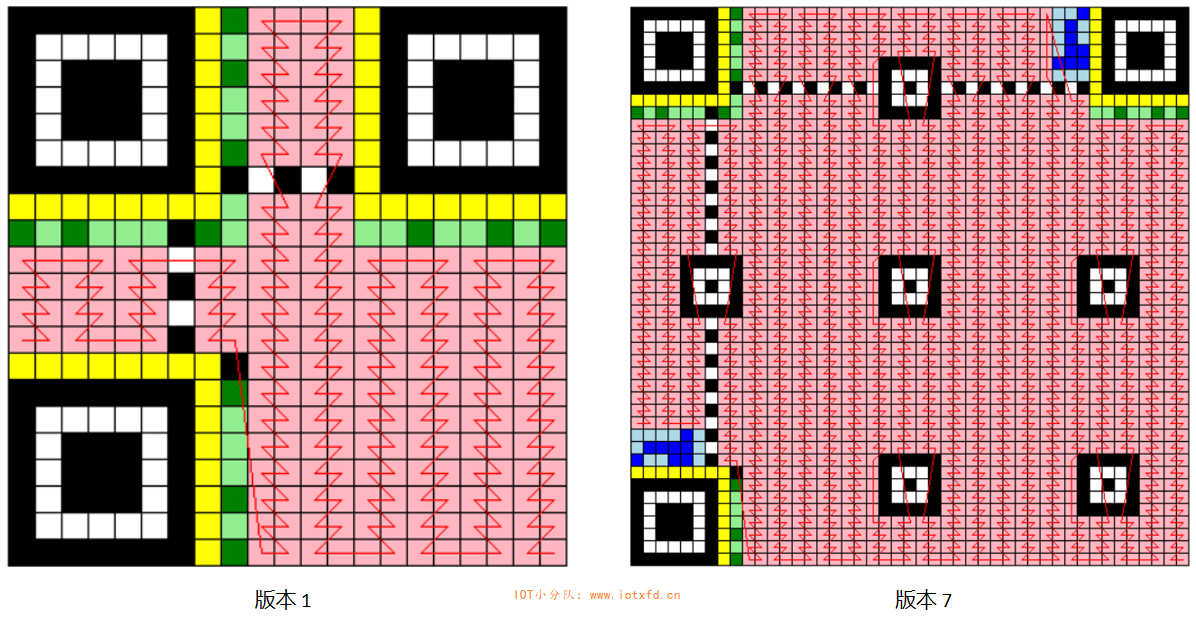This erratic pace gods, ecstasy ah! From the lower right corner to start, with an uninterrupted extension of the line until the end of the lower left corner, the final data stream from left to right, in the direction of this line of modules arranged in a pink encountered along the way, the complete arrangement of symbol characters . I believe we understand at a glance. The reason I want to do this walk route drawing functions, one is hand-painted two pictures too painful, on the other hand also take place in order to facilitate verification algorithm errors.

[Example 4] 1 Continued: symbol character arranged

【例 1 续 2】中我们生成了最终的数据码字为：
0x10 0x20 0x0C 0x56 0x61 0x80 0xEC 0x11 0xEC 0x0E 0x9D 0x02 0xC8 0xC2 0x94 0xF3 0xA7 0xAD 0x8D 0xE2 0x0A 0xF4 0xA5 0x2B 0xAC 0xDF

Now it can finally be filled in accordance with the previous line in the coding region. Results as shown below: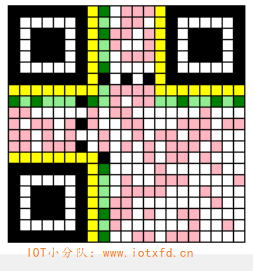Figure in Pink module we just fill in the data. We can finally celebrate, and you can relax, but you can not end wine! It is something else!

QR code if a large area of ​​blank or black block occurs, leading to difficulties in identifying the scanner. To make QR pattern looks as messy, and as far as possible to avoid the bitmap position detection pattern of 1011101, the need for a mask pattern QR operation, the following steps:

The mask pattern is not used for the function and format graphics: finder pattern, positioning pattern, a correction pattern position detection pattern separators, format information and version information.

Data codeword XOR operation performed after the mask pattern is drawn.

The results for each part of the pattern undesired scoring to evaluate the results.

Select the graphic lowest score.

The following table gives the conditions and with reference to the mask pattern generated mask pattern. A mask pattern is formed by those conditions that the coding region (not including format information and version information) is defined as the true dark modules generated. Condition shown, the position of the i-th row represent a module, the module j represents the position of the column, (i, j) = (0,0) representing a symbol in the top left corner position.

condition

000 (i + j) mod 2 = 0
001 i mod 2 = 0
010 j mod 3 = 0
011 (i + j) mod 3 = 0
100 ((i/2)+(j/3)) mod 2 = 0
101 (i × j) mod 2 + (i × j) mod 3 = 0
110 ((i × j) mod 2 + (i × j) mod 3) mod 2 = 0
111 ((i × j) mod 3 + (i + j) mod 2) mod 2 = 0

The following figure shows the appearance of all the mask pattern: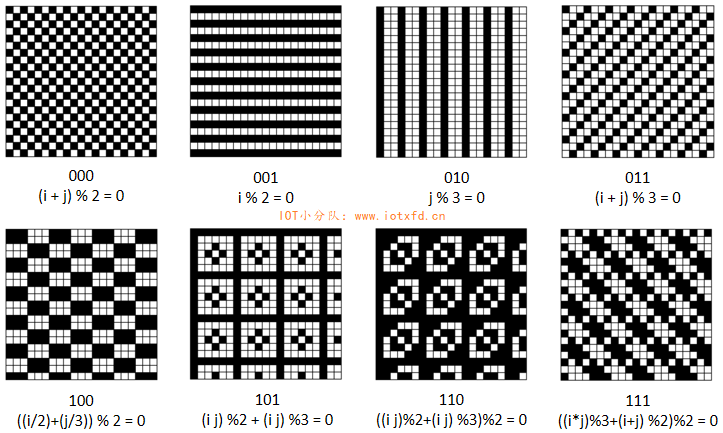Here is the effect after the mask, we can see the entire data of the mask becomes more fragmented.